## Pages

### Crash Course in Quantum Computing Using Very Colorful Diagrams

Almost everything you need to know about Quantum Computing explained using very intuitive drawings…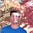Rishabh AnandFollow
Mar 16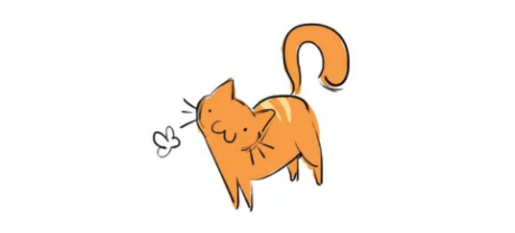Have a quantum cartoon

Quantum Computing is a relatively new, up-and-coming field that smashes the concepts of Quantum Physics, or the study or very small things, and Computer Science.

In this article, I’ll introduce you to the concepts that drive this young, growing field with intuitive analogies and as always, colorful sketches and drawings.

Note: I won’t be covering the math and vector notations in this article for simplicity purposes. If interested in reading such an article, drop a comment below or feel free to contact me via Twitter!

Information representation in classical Computer Science

Classical CS involves the flow and manipulation of bits (binary digits) — the basic units of information in a computer. It can hold the value of either 1 or 0, not both at the same time.

The 1 or 0 usually correspond to the charge of an atom on the hardware, which represents the true/false or on/off states in a computer.

The change of these charges results in the flipping of bits from 1 to 0 or vice versa, allowing information to flow from one location in the memory to another.

Traditional bits are just charges on computer hardware that store information depending on their charge.

Representing information in Quantum Computing

In a traditional computer, information is represented using traditional bits that can only possess a value of 1 or 0 and not both at the same time. In Quantum computers, we represent information using Qubits (quantum bits).

We can represent Qubits using the bra-ket notations: |0⟩ or |1⟩, pronounced ‘ket 0’ and ‘ket 1’ respectively.

Well, in Quantum Computing, the Qubit can have the values of both 1 and 0 simultaneously because of a process called Superposition.
The curious case of Superposition

Superposition refers to a phenomenon where a particle or entity can exist in two states at the same time. It can exist as two things or exist in two different positions. For instance, let’s look at the Schrodinger’s Cat paradox.

If you lock a cat inside a soundproof, inescapable bunker with some explosive material that may likely explode, the cat is in a Superposition of both deadand alive because you don’t know if the material really blew up or not (probably because the bunker is soundproof).

There is a 50–50 chance of the explosive material either blowing up and killing the cat or staying as is. This is taken from the Minute Physics video on Quantum Superposition

The cat is technically both dead and alive so long as you don’t open the bunker and check.

However, a quirky situation arises when an observer actually checks the state of an object in Superposition. In this case, reality collapses to one single outcome and it cannot exist in multiple states at the same time anymore. Similarly, if you can’t hold it in anymore and open the bunker, you may see that the cat is dead.
Guess what? Your curiosity killed the cat…

Or, the cat could still be alive when you check. When an observer (you, in this case) observes an object that was initially in Superposition, they will only see one of n-number of outcomes unfold in front of their eyes. As mentioned before, reality collapses to one of the outcomes.

How is this related to Quantum Computing?

A Qubit can exist in a Superposition, carrying the value of both 1 and 0 at the same time. This opens up a whole new world of possibilities for solving complex problems that require lots of computational power.

Qubits can exist in 2 possible states until observed (which causes reality to collapse to one of the states)

In order to manipulate fundamental bits, they must be transformed by logic gates that either flips bits to another value or let them remain as they are. Some famous examples of logic gates include AND, OR, NOT, XOR and NAND.

They take pairs of bit values and transform them to either a 1 or 0 depending on the functionality of the gate.

An example of a NAND gate that takes in pairs of similar bits and returns the flipped bit

Quantum Logic Gates

To transform Qubits, we pass them through Quantum Logic Gates (or Quantum Gates) that maps the existing state of a Qubit to another state based on the functionality of the gate.

This may sound vague, but Quantum Gates are practically classical logic gates that act on Qubits instead of traditional bits. Quantum Gates can invariably perform all the actions that standard classical logic gates can.

Let’s look at a few examples of Quantum Gates:

Perhaps the most common, the Hadamard Gate takes in a single Qubit as input and outputs a Qubit that has a 50–50 chance of either being a 1 or a 0.

In simple mathematical terms, there is an equal probability of the Qubit collapsing to a 1 or 0. This in itself is a Superposition where the output Qubit has two possible states until we observe it or use it in further calculations.

The Hadamard Gate is represented by an ‘H’ in a box and can be added to a circuit multiple times to output a bit that has an equal chance of being a 1 or 0.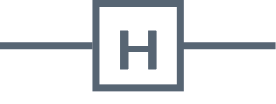The Hadamard Gate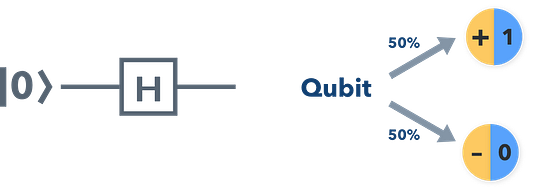There’s a 50–50 chance of the Qubit collapsing to either a 1 or 0
Pauli-X Gates

Named after Wolfgang Pauli (awesome name by the way), the Pauli-X Gate takes in a single Qubit. It is essentially the equivalent to the NOT Gate which negates the bits and flips them. It performs a bit flip on the Qubits. It maps/changes a |0⟩ to |1⟩ and |1⟩ to |0⟩.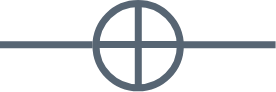The Quantum equivalent of the NOT Gate — The Pauli Gate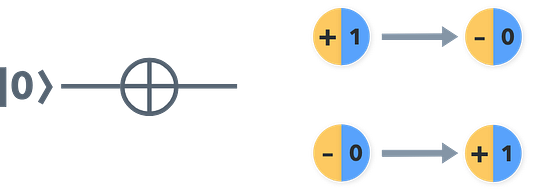The Pauli-X Gate performs a bit flip and negates the Qubits
Controlled-NOT Gate

The Controlled NOT gate is also called the CNOT Gate. This takes in 2 Qubits and only flips the second Qubit from a |0⟩ to |1⟩ and |1⟩ to |0⟩ if the first Qubit is |1⟩. Otherwise, it leaves it unchanged.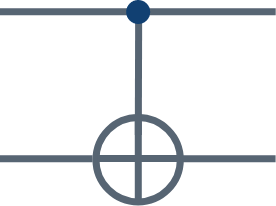The CNOT or Controlled-NOT Gate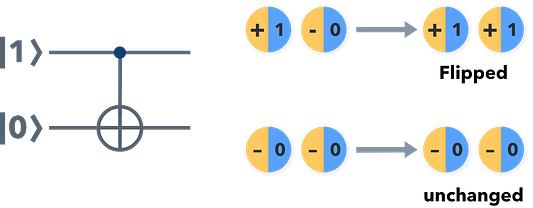The CNOT gate only flips the second Qubit if the first Qubit is |1⟩

Measurement

Measurement is a special type of operations done on Qubits at the end of a circuit to get the final values of the Qubits after the operations have been conducted.

Compared to the gates above, Measurement is irreversible and hence, is not a Quantum Gate. I mentioned earlier that when an observer looks at something that was initially in a Superposition, reality collapses to one of the outcomes.

Similarly, the Measurement function is the observer and takes the collapsed Qubits’ values and puts them together as the final output bits that are either 1 or 0 strictly.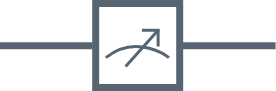The Measurement function is represented as this symbol in a Quantum Circuit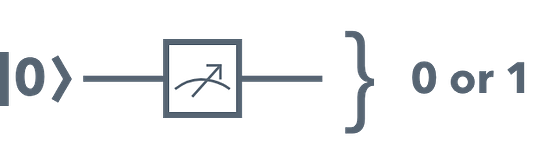The Measurement function takes in a Qubit and observes it to collapse its state to either 1 or 0.

Representing Quantum models

Models are what physicists use to simulate and observe what happens when you poke the universe in a certain way. They help a great deal when it comes to visualization.

Similarly, Quantum models represent situations that deal with numerous Quantum phenomenon (such as Superposition, for instance).
How do we represent a mathematical quantum model on a computer?

We do so by using Quantum Circuits. Circuits represent computational processes in which Qubits are passed through Quantum Logic Gates that transform Qubits from one state to another depending on their type/functionality.

To build a Quantum Circuit, we need a combination of Quantum Logic Gates and Qubits through time to get the desired outcome. To visualize what a Quantum Circuit looks like, take a look at the figure below.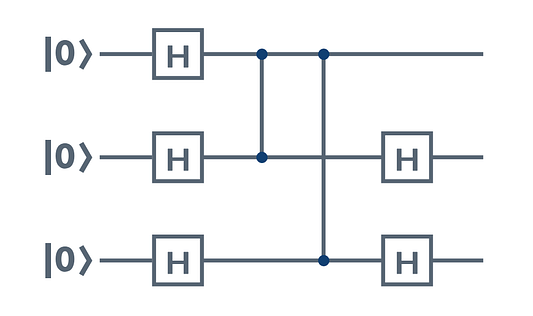The figure shows three Qubits, all with states |0⟩ or ket 0. The H in the boxes represent Quantum Logic Gates (H is the Hadamard gate).

In this figure, we can see the Qubits going through a Hadamard Gate each. The second and third Qubits go through another Hadamard Gate each.

By putting together these Quantum Gates and Qubits in a Circuit, you can visualize the flow of information through the Circuit.

In a nutshell

In this article, I tried to summarise the seemingly-difficult theory behind Quantum Computing. Quantum Computing is an up-and-coming field that has a very small community. I’ll be covering the math and vector notations in upcoming articles on Quantum Computing.

If you want me to cover anything else, drop a comment below or reach out to me on Twitter or LinkedIn. Soon, I’ll be taking you through making your own Quantum Circuit in simple Python (Yes. I said Python).

If there are any mistakes I’ve made, please do let me know (I’m learning too!).

Until then, see you in the next one!

https://towardsdatascience.com/quantum-computing-with-colorful-diagrams-8f7861cfb6da